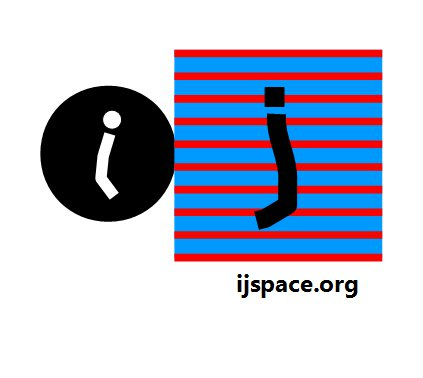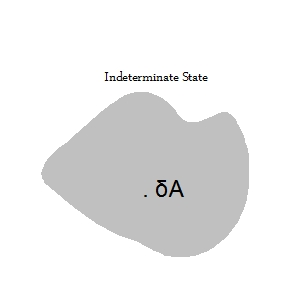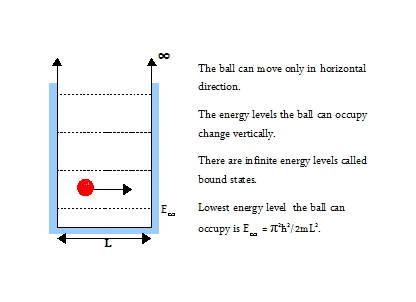Home

Summary: We get ourselves a toy in j-space based on the ideas from Quantum Mechanics.  The particle in a box and the extreme shallow-well problems are discussed.We have a dilemma.  We had set the origin in discrete measurement space as a reference described by an observer of capability equal to the capability of the observer making measurements.  The measurements themselves are set to be PE1j measurements.  The observers making measurements have limited capability defined as [0j, ∞j ].  Therefore the events outside the capability of the observers can be regarded as indeterminate.  What does it mean by an indeterminate state?  Let us try to answer that.  First we describe a methodology.

Consider dropping a small pebble into the pond in Chicago.  The action will cause a ripple we can measure.  Now if the pond is large enough, which actually it is, the ripple will not change anything really.  We call it an infinitesimal perturbation.  We also know that the contents within pond can be considered uniform, therefore the results obtained from our infinitesimal action and subsequent measurements will be applicable everywhere in the pond.  And we will be able to predict the behavior of its contents or a similar system to a much higher accuracy.

In physics we have similar methodologies to analyze a given system.  For example the principle of virtual work allows the study of a physical system in equilibrium.  We displace a system in equilibrium by an infinitesimal amount.  Since the displacement is infinitesimal, the total work sums up to zero hence the name virtual work.  We can thus obtain the relationship between the different components of the internal forces maintaining the equilibrium state.  In Quantum Mechanics we apply a small perturbation to a system in its lowest energy state and study its behavior in its excited states.

## Indeterminate StateLet us consider the indeterminate state and introduce a action δA in it by the application of an infinitesimally small measurement force.  The shape of the indeterminate state is deliberately drawn not to be symmetric.  We note the application of the measurement force by an observer immediately puts the observer in discrete j-space.

The action
δA will introduce the complimentary simultaneous states, one of them will allow a measurement to take place other will not allow a measurement to take place in the discrete measurement space.  Combined together they will result in an indeterminate state.  We just separate them by an infinitesimal amount below the observer's measurement threshold.Both of these states will behave as sources for the observer in the discrete measurement space.  We have introduced a perturbation which has restricted the space within discrete measurement space into regions where measurement can be made and measurements can not be made.  Or in other words the regions where a particle's existence is measured or not measured.

Further for an observer with low efficiency the required measurement force will be much larger and resolution between the both types of regions will be much coarser.  This situation is analogous to the collider schemes where to access information about fundamental particles, a very large measurement force and hence a much higher energy input is required.

An observer who can measure states created by only one of either "continuum" or "nil" sources but not both, is considered a zero-efficiency observer.

We note the concept of the region where the particles can not be measured is different than conventional vacuum state which is based on source and sink concept.  In this case the particles are physically removed to create vacuum which is essentially a classical thermodynamic system.

## The Particle in BoxIn Quantum Mechanics we have a concept of the particle in a box, which means a constraint that can not be violated.  The example is a ball constrained to move along a line, or a knot which can not be opened.

Suppose that the box can be opened then the structure is called a "potential-well". In a box we have infinite bound states.  In a well there are finite number of bound states.  The number of bound states in a well, are dependent on the depth of the well Uo and how it compares to E
. If Uo >> E then there are large number of bound states.

As it happens that when Uo is less than
E there is a single bound state.  In the case when Uo << E which is known as "shallow-well", the energy of the bound state is approximately  0.68Uo.

## Application to j-space

So what has that got to do with discrete measurement space?  Replace the ball with an observer.  If the observer has very low capability, then we have a situation analogous to the particle-in-a-box problem.  There are infinite states which need to be measured and the observer can not complete the measurements or get free.  Completing the measurements is a PE1 event on our abacus.

Again if the observer has infinite capability then the situation is analogous to a shallow-well problem and therefore the observer has to measure only one state to complete a PE1 event.  The Obsi observer is an example of this case.  This is an important point we must remember.  Thus we can define our own prisons based on our respective capabilities.Another way to look at it is that
the continuous i-space is represented by a true point.  The j-space is discrete in nature and can not represent the true point, but a true-point can be approximated in j-space by an infinitesimal box with infinite energy states.  The barrier between the i-space and j-space is an infinite barrier for j-space however it remains a finite barrier for i-space.  It means that a signal from i-space can extend into j-space but opposite is not true.Let us now consider the interface between i-space and  j-space such that capabilities follow the relationship i >> j.  There will be discontinuity at the interface in measured signal values as the extension of i-space δi however small, represents a quantity much greater than j.  At the same time it has a finite value for observer Obsi.

While Obsi measures <t = 0j> event without applying a measurement force, the observer pair in discrete j-space uses the Qbox to measure the < t = 0j> event and its evolution.  We will develop this description next when discussing the entropy, the negentropy and the information in the discrete j-space." You should not be led along blindly."

- Shang-Keng Ma, in Statistical Mechanics, World Scientific.

"...we have to assume that there is a limit to the fineness of our powers of observation and the smallness of the accompanying disturbances..."

- Dirac in The Principles of Quantum Mechanics, Oxford Science  Publications.

Sears and Zemansky, University Physics, Pearson's Education, Inc. is a good starting point for basic quantum mechanics concepts.